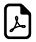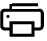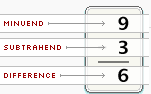# Glossary Used In Basic Math Subtraction## Glossary Used In Subtraction

Operations Vocabulary Explanation Example
Subtraction Minuend The first number in a subtractionSubtrahend Number that is to be subtracted. 2nd no.in a subtraction
Difference Difference is the result of a subtraction problem.

## Subtraction Example1:

3 - 2 = 1 ,

Here, 3 is Minuend

2 is Subtrahend

1 is Difference

### Subtraction Example2:

23 - 2 = 21 ,

Here, 23 is Minuend

2 is Subtrahend

21 is Difference

###### These vocabulary of subtraction is the basic things in general mathematics###More Pages ►

Square & Square Root Calculator

Sigma Notation Calculator

Sum of Square Calculator

Sum of Cube Calculator

Nth Root or Radical Calculator

Exponent Solver

Scientific Engg Notation

Prime Number Factorization